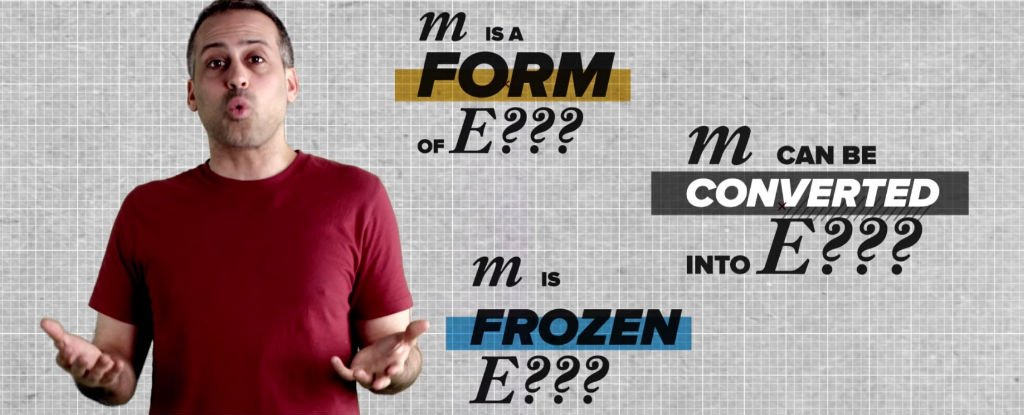Videos# WATCH: Here’s the Real Meaning of E = MC2

Here’s a weird fact for you – a hydrogen atom has less mass than the combined mass of the proton and electron that make it up. Okay, it’s not just hydrogen – ALL elements on the periodic table weigh less than the sum of their parts.

Here’s another mind-bender: if you have two objects are made up of identical constituents, down to their very atoms, those objects will very likely have different masses. Why? That’s all down to the most famous equation in physics, Einstein’s E = mc2.

As the video below by PBS Space Time explains, the mass of something that’s made up of smaller parts does not equal the sum of the mass of those parts.

If you want to figure out its true mass, you’ve got to take into consideration how those various parts are arranged, and how those parts move within the structure of the larger object.

For example, if you gave Einstein two identical stopwatches – and I mean identical down to the atomic level – but only one was fully wound up and running, he’d tell you the moving one had a greater mass than the stationery one.

How does it work?

All that kinetic energy from the moving hands, the potential energy from the springs, and the friction in the gears all add up to extra mass, according to E = mc2.

But just when you thought you’d wrapped your head around the whole ‘potential energy equals extra mass’ thing, remember that hydrogen atom weighing less than the combined mass of its parts?

Turns out, potential energy can also be negative. (╯°□°）╯︵ ┻━┻

How does this work? As the video explains, the fact that the proton and electron are placed relatively close together in the hydrogen atom means their potential energy has been reduced from zero to a minus value.

We get extra kinetic energy from the electron moving around the proton, but even this is not enough to put the two values back to zero. This applies to every single element on the periodic table.

But what if we get really tiny, and look at the sum of parts of protons and electrons – quarks. And where do quarks get their mass from if the Standard Model of Physics says they’re not made up of any parts?

Don’t freak out, the PBS Space Time episode above has all the answers, and will give you a whole new appreciation for the fact that one simple equation has all of this covered.

We don’t say this enough, but thanks, Einstein.

Here’s more on those pesky quarks: